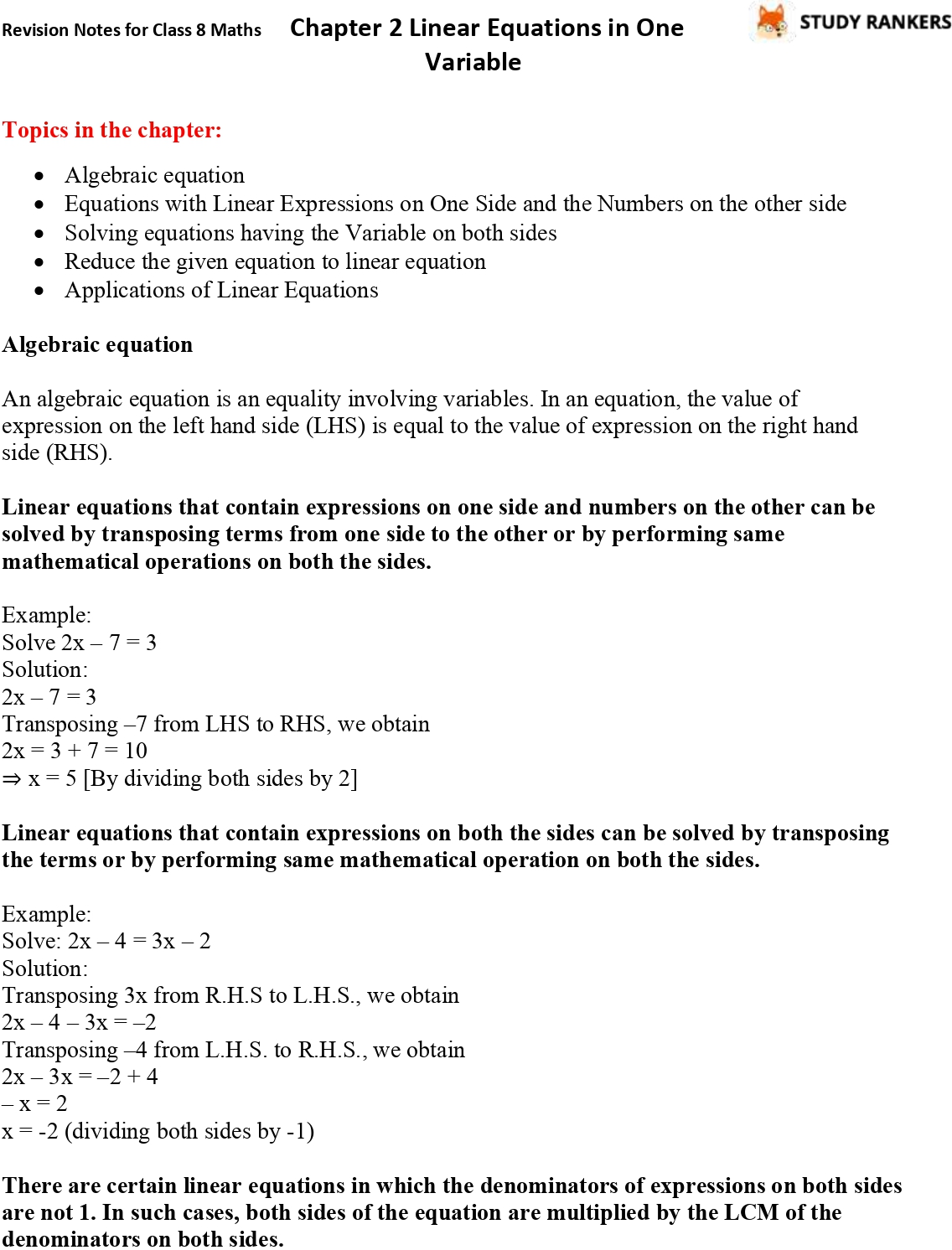## CBSE Revision Notes for Class 8 Chapter 2 Linear Equations in One Variable

Chapter 2 Linear Equations in One Variable Class 8 Revision Notes is provided here which will be useful in knowing about the topics given in the chapter and understanding the basic concepts. These Chapter 2 Class 8 Notes will be also helpful in revising the important topics just before in a precise manner. It will increase your confidence and getting more marks.

The topics in the chapter are:
• Algebraic equation
• Equations with Linear Expressions on One Side and the Numbers on the other side
• Solving equations having the Variable on both sides
• Reduce the given equation to linear equation
• Applications of Linear Equations